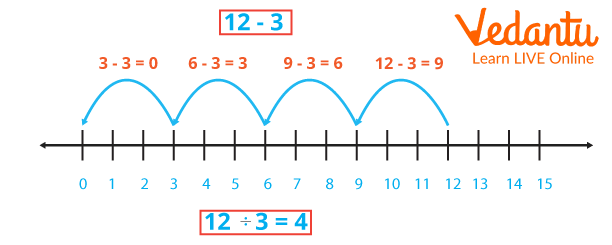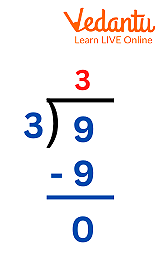Courses
Courses for Kids
Free study material
Free LIVE classes
More

# Division is a Process of Repeated SubtractionLIVE
Join Vedantu’s FREE Mastercalss

## Introduction to Division

One of the four fundamental arithmetic operations, or how numbers are combined to create new numbers, is division. The additional operations are multiplication, addition, and subtraction. The technique of repeatedly subtracting one number from another until the final result is zero is known as division. This method is frequently used and seen when dividing numbers and is an effective technique to teach division to younger grades. To further understand the concept, let's study more about repeated subtraction, how to divide using repeated subtraction, and how to solve a few problems.

## Division is a Process of Repeated SubtractionDivision is the Process of Repeated Subtraction

The act of repeatedly subtracting the same number from a large number until the final result, or remainder, is zero or less, is referred to as repeated subtraction. Another name for this procedure is division. In other words, repeated subtraction is the process of subtracting the same number from the other number until the remainder is zero or until it is smaller than the original number. This operation can also be written in the form of division. As in an illustration $12 - 3 = 9,9 - 3 = 6,6 - 3 = 3,3 - 3 = 0$ . This indicates that the number has been deducted five times, and the division form is$12 \div 3 = 4$. Thus Repeated Subtraction is Called Division.

## Dividing 1 Digit Number

• Take the dividend's first digit starting from the left in step one. Verify that this digit exceeds or is equal to the divisor.

• Next, divide it by the divisor, and write the result as the quotient on top.

• Subtract the outcome from the digit, and then write the difference below.

• Decrease the dividend's subsequent digit (if present).

• Carry out Step 4 again.

From the given image, we can understand how to divide 1 Digit Number.Example of How to divide 1 Digit Number

## Division by Repeated Subtraction

Similar to how repeated addition is used to solve multiplication problems, repeated subtraction can be used to address difficulties with division. Dividend, divisor, quotient, and remainder are the four components of division that are taken into account when dividing any integer.

The procedures for division by iterative subtraction are as follows:

• Take note of the dividend and divisor. Dividend and divisor both refer to an amount that must be divided; the dividend is the amount that the divisor divides.

• Subtract the divisor once again using that number after obtaining the difference.

• If you don't get a result that is less than the divisor or zero, keep trying to subtract.

• The quantity of times the subtraction procedure is finished is referred to as Quotient.

• The remainder is the amount that remains after subtracting a number.

## Examples for Dividing 1 Digit Number

Example 1: Use the repeated subtraction method to divide 120 by 15.

120 divided by 15 is the dividend.

Repeatedly subtract 15 from 120.

$\begin{array}{l}120 - 15 = 105\\105 - 15 = 90\\90 - 15 = 75\\75 - 15 = 60\\60 - 15 = 45\\45 - 15 = 30\\30 - 15 = 15\\15 - 15 = 0\end{array}$

Here, 15 is removed eight times from 120, leaving 0 as the remainder. Thus, the quotient is $120 \div 15 = 8$ , which is 8.

Example 2: Bella needs to provide 8 apples to each of her friends out of the 48 she has on hand. How many buddies can you divide 48 apples equally among?

Given that Dividend = 48 and Divisor = 8,

Using the method of repeated subtraction,

$\begin{array}{l}48 - 8 = 40\\40 - 8 = 32\\32 - 8 = 24\\24 - 8 = 16\\16 - 8 = 8\\8 - 8 = 0\end{array}$

Therefore, 48 is taken away six times from 8, leaving behind 0.

Bella can therefore distribute 48 apples among her six buddies.

Example 3: Divide 60 by 10 by the process of Repeated Subtraction.

For $60 \div 10$,

$\begin{array}{l}60 - 10 = 50\\50 - 10 = 40\\40 - 10 = 30\\30 - 10 = 20\\20 - 10 = 10\\10 - 10 = 0\end{array}$

Thus, $60 \div 10 = 6$.

Last updated date: 02nd Oct 2023
Total views: 68.1k
Views today: 1.68k

## FAQs on Division is a Process of Repeated Subtraction

1. What three types of division are there?

According to the degree of difficulty, there are three different types of division methods. These include the bus stop method, long division method, and the chunking method, also known as division by repeated subtraction.

2. How Can You Subtract Multiple Times?

Repeated subtraction is the process of repeatedly subtracting the dividend from the divisor up until the remainder equals zero or less.

3. Write the significance of repeated subtraction.

It's crucial to repeatedly subtract while teaching younger kids how to divide. It serves as a more straightforward and understandable introduction to division.